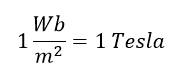# What is Magnetic Flux

## Magnetic Flux:

Magnetic flux is the measurement of the total number of magnetic field lines are passing through a surface or given area. The magnetic flux through a closed surface (such as a ball) is always zero. The magnetic field is analogous to electric current I in an electric circuit. The CGS (Centi Meter Gram system units) unit of magnetic flux is Maxwell. But The SI unit of magnetic flux is the Weber (Wb).

Magnetic flux is sometimes used by electrical engineers designing systems with electromagnets or designing dynamos.  Example: generator, motor, Electromagnets, etc.

Get More Electrical Concept And Interview Questions By Using This Link

More generally, the magnetic flux can be found using the vector dot product. Net numbers of lines passing through the surface are called magnetic lines of forces.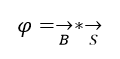If the magnetic field is constant than the magnetic flux passing through a surface (S) is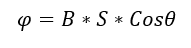where
B – the magnitude of the magnetic field (generally Magnetic field density)
S – Surface area
θ – angle between the magnetic field lines and perpendicular distance normal to the surface area
Magnetic flux for closed surface

Also Know About:   What is Motor? How Motor Works With Graphical Presentation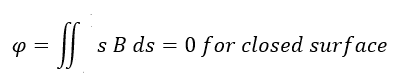Magnetic flux for open surface..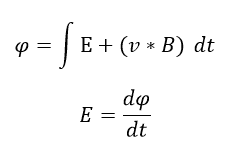Where
E – electromotive force.
v – velocity of the boundary
E – electric field
B – magnetic field
ø –  magnetic flux through the open surface
dl – infinitesimal vector element

The magnetic flux passes through a closed surface is always equal to zero, but in the open surface, it is not zero.

How to measure magnetic Flux: The magnetic flux is measured by Weber meter.

## Magnetic Flux Properties:

Main article: Magnetic field

• Magnetic Field lines attempt to form closed loops from pole to pole. These lines are not discontinuous as of electric field
• They never intersect each other.
• The field starts from south Pole and ends in north pole (within the body)
• Outside the body of magnetic direction of lines is from North Pole to South Pole (outside of the body)
• Tangent to field line at any point gives the direction of magnetic field at that point.
• Their strength decreases with increasing distance from the poles. The strength of field can be varied in accordance with the amount of current flowing in the conductor around the coil.
• Unlike poles attract (North and South), Same Poles (North & North or South & South) rebel
• Field lines are high concentrate in Pole corner side and low concentration in center of the body.
Also Know About:   What is Voltage Drop Allowable Limit and Calculation

## Magnetic Flux Density:

The total number of magnetic field lines (magnetic flux) are passing through a unit surface area perpendicular to the magnetic field is called magnetic flux density.

Here φ is the magnetic flux, surface area A in meterthe magnetic field density B (generally represent magnetic flux density by capital letter B).
Get More Electrical Concept And Interview Questions By Using This Link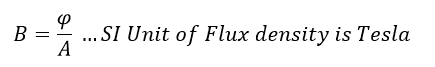SI units of Magnetic field density is Tesla. Also it is measured by Wb/m2